§ 5   Nonlinear integral equations

[ Integral and Linear Operators ]   Consider the expression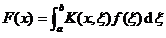For a given kernel K ( x , ξ ), each function f ( x ) has another function F ( x ) corresponding to it. This correspondence is called an integral operator, denoted as K , namely

F=Kf

The set of those functions f such that the function F = Kf exists is called the domain of operator K.

If operator K satisfies the condition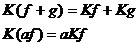( a is a constant)

Then K is called a linear operator.

[ Bounded operator and its norm ] If there is a constant M , for all functions f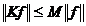Then K is called a bounded operator, where it represents the norm ( modulus ) of the function f . The largest lower bound of all M that makes the above inequality true is called the norm of operator K , denoted as , it can also be defined as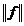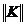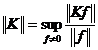Bounded operators have the following properties:

If K 1 and K 2 are bounded operators, then K 1 K 2 is also a bounded operator.

2° If the  function K ( x , ξ ) is continuous for all x , ξ , on the finite square k 0 ( axb , aξb ) , then by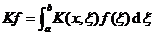The defined operator K is a bounded operator.

If on the infinite interval [ a, b ] , the function K ( x, ξ ) satisfies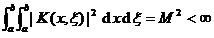then byThe defined operator K is a bounded operator.

[ Theorem of existence of solutions to nonlinear integral equations ] Consider an integral equation of the form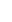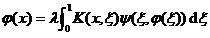(1)

The methods of solving linear integral equations in the previous sections are not applicable to nonlinear integral equations. The existence theorems of just a few solutions are listed below.

Theorem 1 assumes that K ( x , ξ ) is continuous for all x, ξ on the unit square k 0 (0 x1,0 ξ1) , let ô K ( x, ξ ) ôC ( C ( C is a constant ) , for all ξ on the unit square k 0 , t is also continuous, and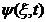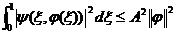( A is a constant )

It is also assumed that the Lipschitz condition is satisfied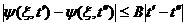where B is a constant independent of ξ . At that time , the integral equation (1) has a unique solution in L 2 [0,1] * .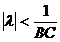Theorem 2 assumes that K ( x, ξ ) is continuous with respect to all x, ξ on the unit square k 0 , let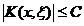( C is a constant)satisfy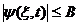( B is a constant)

and for any ε > 0 , there is δ = δ ( ε ) such that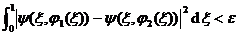( at the time )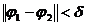in the formula . Then at that time , integral equation (1) has at least one solution in L 2 [0,1] * .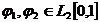Theorem 3 assumes that K ( x , ξ ) and are both continuous functions of their independent variables, let S be L 2 [0,1] satisfying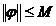( M is a constant)

the whole of the function . assumed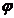( C is a constant)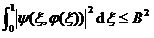(everything )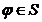And for any ε > 0 , there exists δ = δ ( ε ), such that( at the time )At that time , the integral equation (1) has at least one solution in S.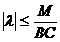The condition of this theorem requires that K ( x , ξ ) be continuous, and in fact it can be shown that the same result is obtained as long as the kernel K ( x , ξ ) is square-integrable.

Theorem 4 assumes that the conditions stated in Theorem 3 are satisfied, and let K ( x, ξ ) satisfy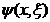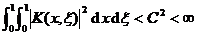At that time , the integral equation (1) has at least one solution in S.* represents the entirety of all square-integrable functionson the interval